Mobile QR Code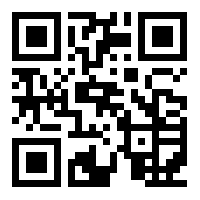1. (Faculty of Interdisciplinary Science and Engineering in Health Systems, Okayama University / Okayama, Okayama Prefecture, Japan {y-tarutn, yokohira}@okayama-u.ac.jp)
2. (Graduate School of Natural Science and Technology, Okayama University / Okayama, Okayama Prefecture, Japan)
3. (Faculty of Natural Science and Technology, Okayama University / Okayama, Okayama Prefecture, Japan fukusima@okayama-u.ac.jp )

Internet of things, Reinforcement learning, Consensus builder

## 1. Introduction

In recent years, IoT devices have become widespread. Such devices are used to collect a variety of information and to control actuators. By controlling actuators based on the collected information, it is possible to achieve various purposes. An energy management system (EMS) is one use case. EMSs visualize power consumption and control actuators to reduce power consumption. Although EMSs have been deployed in buildings, factories, and data centers, with the spread of IoT devices, EMSs in living environments have also been proposed [1,2]. In such environments, an EMS needs to consider the effect of that control on user satisfaction. For example, some users may be sensitive to cold, while others are sensitive to heat. Thus, air conditioner settings determined by an EMS in these situations may result in some people feeling uncomfortably hot, while others feel uncomfortably cold. Increasing user satisfaction through device control is therefore a challenge.

In , we proposed a device control method based on consensus building. In the conventional method, a user stress model is developed for consensus building by collecting experimental data. This model determines individual user stress resulting from the environment. In this study, we assume that stress models for all users in the target environment are developed. Thus, we focus on the influence of user satisfaction by changing the temperature and light color. The conventional method calculates device parameters to minimize power consumption under the constraint of user satisfaction. However, the conventional method does not increase user satisfaction because it is a constraint; it needs to be treated as an objective function to increase user satisfaction.

In this paper, we propose a new consensus building method to reduce power consumption and increase user satisfaction. Using an exhaustive search for the values of device parameters incurs calculation overhead. The proposed method uses reinforcement learning to solve this problem. Reinforcement learning does not require learning from a data set, unlike supervised learning. Therefore, it is suitable for problems where it is difficult to prepare a data set.

The remainder of this paper is organized as follows. Section 2 describes the conventional method based on consensus building. Section 3 describes our proposed method for consensus building. Evaluation results are described in Section 4, and Section 5 offers the conclusion and describes future work.

## 2. Conventional Consensus Building

### 2.1 Target Environment

Fig. 1 shows an overview of the proposed energy management system, which includes the user platform (UP) and the application service platform (ASP). The UP is a living environment such as an office. This platform includes various control devices and sensors in which the sensors transmit data to the ASP through the Internet. The ASP controls devices through messages based on the collected sensing data.

##### Fig. 1. Overview of the energy management system in this study.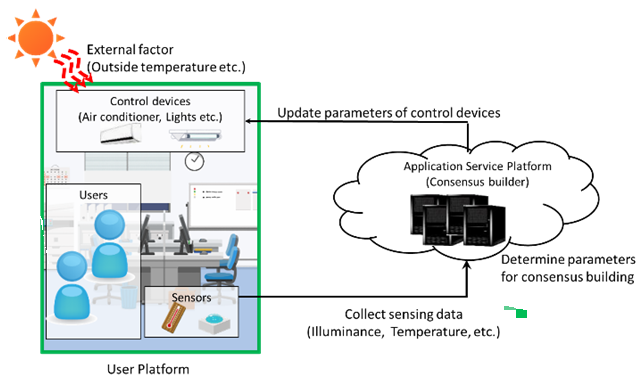In our study, the EMS calculates device parameters to increase user satisfaction in the UP. User satisfaction is affected by the room environment (e.g. temperature, color of the light, etc.) [4-6]. For example, the authors in  reported that user stress can be decreased by changing light color. Therefore, we focus on changes in the user’s stress due to changes in room temperature and illumination color.

### 2.2 User Stress Model

Some researchers proposed methods for detecting user stress through sensing data [7-12]. They showed that stress can be detected without directly asking users about their preferences and satisfaction ratings. However, these methods require users to wear devices, such as electrocardiographs or brainwave meters, to collect biodata.

In , we used a user stress model for detecting the user’s reaction to each control device parameter. The conventional method calculates the parameter values for consensus building based on user models.

In this study, we use heart rate variability (HRV), which is commonly used as a stress index . In previous research, each user stress model was developed through experiments by using environmental and biological data. In our experiment, we collected the HRV in the subjects under various room temperatures and illumination colors. Fig. 2 shows an example of the user stress model, in which the horizontal axis is the room temperature and the vertical axis is light color. The colored bars represent the acceptable temperatures for users with each color of light. In the figure, the double circles, the circles, and the crosses indicate good, normal, and bad conditions, respectively. As shown in this figure, we classify a user’s stress into three categories based on HRV: good, normal, and bad.

##### Fig. 2. Example of the user stress model.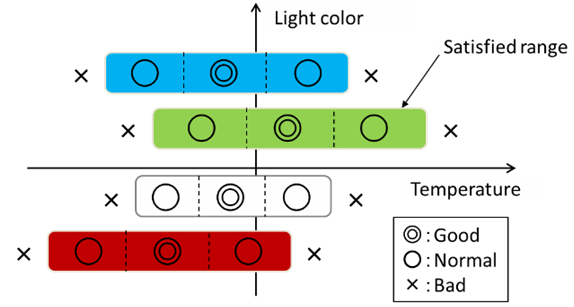### 2.3 Conventional Method

Next, we describe formulation of the problem under the conventional method. The power consumption of each device depends on its parameters (e.g. temperature and mode of the air conditioner, brightness and color of the light, etc.) and the environment of the devices. For example, the power consumption of an air conditioner depends on the values of device parameters, the room temperature, and the outside temperature. Therefore, the power consumption of device $\textit{j}$, $p_{j}$, is defined by Eq. (1):

##### (1)
$p_{j}=f\left(a_{j},s_{j}\right)\,\,\,\left(1\leq j\leq M\right)$

where $a_{j}$ and $s_{j}$ form sets of device parameters and sensor values, respectively, related to device $\textit{j}$, and $\textit{M}$ is the number of devices.

Next, the user stress level of user $\textit{i}$, $u_{i}$, is defined with Eq.(2):

##### (2)
$u_{i}=\left\{\begin{array}{l} 1\left(\mathrm{Good}\right)\\ 0\left(\mathrm{Normal}\right)\\ -1\left(\mathrm{Bad}\right) \end{array}\right.\,\,\,\,\left(1\leq i\leq N\right)$

where $\textit{N}$ is the number of users. The problem solved by the conventional method is expressed in Eq. (3):

##### (3)
$\max \sum _{j=1}^{M}R\left(p_{j}\right)$

Subject to $u_{i}\geq 0\left(i=0,1,\ldots ,N\right)$

where $R\left(p_{j}\right)$ is the reward from power consumption as determined by $p_{j}$. A lower power consumption gives a greater reward. The conventional method calculates the values of device parameters, so the reward from power consumption is maximized under the constraint that user stress is either good or normal.

Eq. (4) indicates the calculation overhead under the conventional method:

##### (4)
$N\prod _{j=1}^{M}d_{j}$

Here, $d_{j}$ is the parameter for the number of degrees for device $\textit{j}$. Eq.(4) indicates that the calculation overhead is the product of the number of users, the number of devices, and the degrees of devices. So, for each increase of $\textit{N, M}$ and $d_{j}$ have a greater effect on overhead. Therefore, it is difficult to calculate the values for device parameters by using an exhaustive search.

## 3. The Proposed Device Control for Consensus Building

### 3.1 Problem Formulation

In this paper, we propose a method where the rewards from both power consumption and user satisfaction are considered. Thus, power consumption and user satisfaction are objective functions. By including user satisfaction as an objective function, we search for the device parameters that maximize user satisfaction and power consumption rewards. Thus, the problem to be solved in this study is shown in Eq. (5):

##### (5)
$\max \left(\alpha \sum _{i=1}^{N}R^{u}\left(u_{i}\right)+\beta \sum _{j=1}^{M}R^{p}\left(p_{j}\right)\right)$

Here, $R^{u}\left(u_{i}\right)$ is the reward from user satisfaction as determined by $u_{i}$, $R^{p}\left(p_{j}\right)$ is the reward from power consumption as determined by $p_{j}$, and ${\alpha}$ and ${\beta}$ are weights of the rewards from user satisfaction and power consumption, respectively. As described in Section 2, user satisfaction calculated from the user model is classified into three categories. In addition, $R^{u}\left(u_{i}\right)$ is adjusted based on the number of users who feel bad. In other words, when more users feel good, the reward is higher, and when more users feel bad, the reward is lower. Power consumption reward $R^{p}\left(p_{j}\right)$ is calculated from the power consumption of devices in the environment. The power consumption reward is set so that lower power consumption increases the value. In addition, the priority of user satisfaction and power consumption rewards can be adjusted by changing the weights.

The conventional method uses an exhaustive search to calculate the values of device parameters, as described earlier. If we use the same approach to the problem in Eq.(5), a large calculation overhead is required because it is necessary to search for all control values to consider both power consumption and user satisfaction. Therefore, in this paper, we propose a new method that applies reinforcement learning.

### 3.2 Applying Reinforcement Learning for Consensus Building

Reinforcement learning is machine learning that maximizes rewards through trial and error. Fig. 3 shows the process, which consists of two parts: the agent and the environment. The agent is decides what action to take in response to a certain condition. The environment evaluates the action of the agent.

##### Fig. 3. The process in reinforcement learning.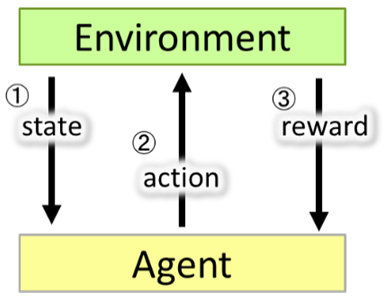In reinforcement learning, the agent’s learning progresses so the reward is maximized by the interactions between agent and environment. The agent and environment exchange three elements: state, action, and reward. The state represents current information about the environment. The action represents the kind of behavior the agent takes in the environment. The reward represents the evaluation of the agent’s action based on the state in the environment. The action value function calculates the expected value of the total reward (TR).

In Q-learning (a typical reinforcement learning method), the action value function, Q($s_{t}$, $a_{t}$), is updated as follows:

##### (6)

Q($s_{t}$, $a_{t}$) ${\leftarrow}$ Q($s_{t}$, $a_{t}$) + ${\alpha}$${\Delta}$Q

${\Delta}$Q =$r_{t}$ + ${\gamma}$ · max Q($s_{t+1}$, $a_{t+1}$) ${-}$ Q($s_{t}$, $a_{t}$)

where $s_{t}$ is the state at time $\textit{t}$, $a_{t}$ is the action at time $\textit{t}$, $r_{t}$ is the reward at time $\textit{t}$, ${\alpha}$ is the learning rate, ${\Delta}$Q is the error between the current output and the target value, and ${\gamma}$ is the discount rate. Action value function Q converges to the optimal action value function via Eq. (6). As a result, the optimal action is selected under Q-learning.

The problem of the Q table is that as the number of dimensions for states and actions increases, the size of the Q table becomes enormous and overhead increases. An approach to this problem is to apply deep learning. By approximating the action value function with a deep neural network (DNN), reinforcement learning can be implemented without preparing a Q table. This is called deep reinforcement learning.

Fig. 4 shows the overview of reinforcement learning in the proposed method. From Fig. 4, the state in Fig. 3 corresponds to values obtained from various sensors. Similarly, the action determined by the agent corresponds to the parameter values of all devices installed in the room. In the proposed method, the reward is calculated from the satisfaction levels of all users and the total power consumption by using Eq. (5).

##### Fig. 4. Overview of the proposed method.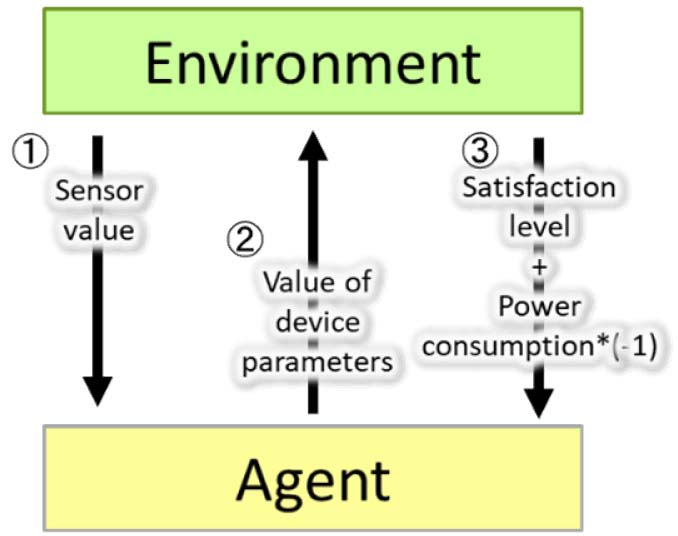## 4. Evaluation

For the evaluation, we constructed a learner via reinforcement learning based on user models and outside temperature data. Next, we obtained values for device parameters by using this learner to calculate the rewards. Then, we evaluated the effectiveness of the proposed method by comparing it to the conventional method and the optimum control (exhaustive search) that maximizes the reward.

### 4.1 Evaluation Environment

#### 4.1.1 The Scenario

In this evaluation, we set the elements of Fig. 4 as follows. First, we used room temperature as a sensor value. The device parameters were the air conditioner setting (ACS) and the lighting. The air conditioner mode was set to cooling, and the temperature range was 20-29 degrees C. In addition, lighting could be individually set for each user and selected from four colors. To simplify the evaluation, we assumed the room temperature was the previous air conditioner setting. The initial room temperature before controlling it was 25 degrees C.

Power consumption by the air conditioner is much larger than power consumption by the lighting. Therefore, the reward for power consumption is based on the air conditioner setting. In this evaluation, because we used cooling mode, a lower temperature setting means higher power consumption. In addition, the outside temperature affects power consumption by the air conditioner. Therefore, power consumption reward $R_{t}^{p}\left(p_{j}\right)$ is calculated with Eq. (7):

##### (7)
$R_{t}^{p}\left(p_{j}\right)=T_{t}^{s}-D_{t} \\ D_{t}=\left\{\begin{array}{l} T_{t}^{o}-T_{t}^{s}\\ 0 \end{array}\begin{array}{l} \left(\mathrm{if}\,\,T_{t}^{o}>T_{t}^{s}\right)\\ \left(\text{otherwise}\right) \end{array}\right.$

where $T_{t}^{o}~$and $T_{t}^{S}~$are the outside temperature and the temperature setting, respectively, at time $\textit{t}$. In this study, we assumed the action does not affect the future power consumption reward, $R_{t+1}^{p}~ \left(p_{j}\right)$. Therefore, discount rate ${\gamma}$ in Eq. (6) was set to 0 for this evaluation.

We generated 10 user models. In the first evaluation, we considered four cases where five users in each case are randomly selected from the user models. We changed weight value ${\alpha}$ for verification of the tradeoff between user satisfaction reward and power consumption reward. We set weight ${\beta}$ to 3, and weight ${\alpha}$ to 2.5 or 3. We evaluated the proposed method for 10 users. Moreover, in this evaluation, we assumed that all lighting settings are the same (i.e. no individual settings). This is because computational resources were insufficient for this evaluation.

Device control was executed at hourly intervals during the evaluation period of one month. For the outside temperature data, we used August 2018 as provided by the Japan Meteorological Agency .

#### 4.1.2 Parameter Settings

The framework and the learning parameters are shown in Table 1. The number of updates was set to 5000, as confirmed by examining the number of convergences in multiple patterns.

##### Table 1. Framework and learning parameter settings.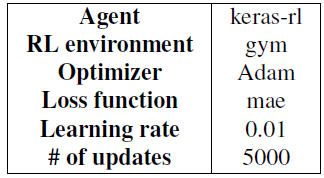### 4.2 Evaluation Results

Figs. 5 and 6 show the results from each method. In each graph, the vertical axis is temperature and the horizontal axis is time. The blue line represents the outside temperatures in August 2018. The orange, green, and red lines represent air conditioner settings under the proposed method, the conventional method, and the exhaustive search, respectively.

From Figs. 5 and 6, the air conditioner settings under the conventional method are constant values for all user patterns. In the conventional method, user satisfaction is treated as a constraint, and the maximum temperature setting within the range of the constraint is selected. Even if the power consumption reward decreases due to an increase in the outside temperature, the temperature setting cannot be changed due to the constraint on user satisfaction. On the other hand, the proposed method treats user satisfaction as part of the objective function. So, the setting can be changed in response to changes in outside temperature. Therefore, almost the same control result is obtained, compared with the exhaustive search.

##### Table 2. Percentage of user satisfaction levels in all periods (${\alpha}$ = 3).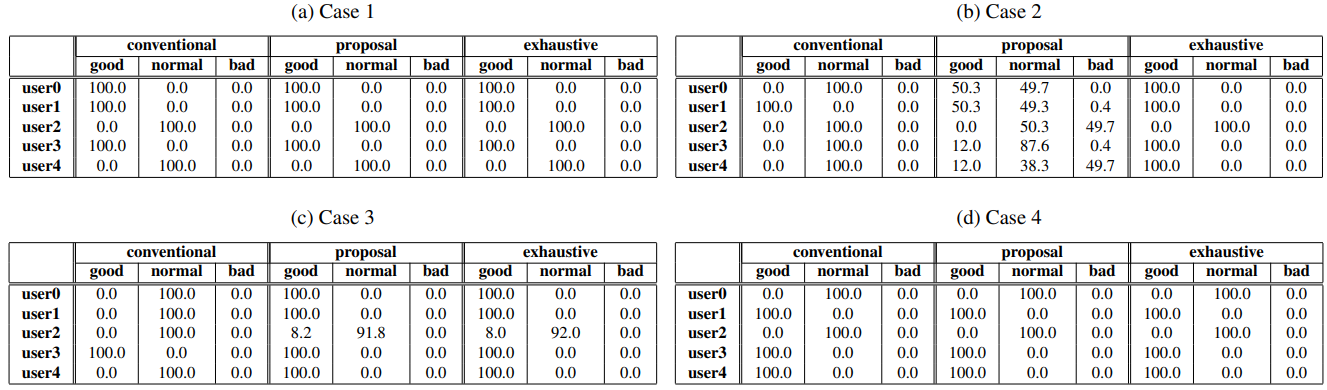##### Table 3. Percentage of user satisfaction levels in all periods (${\alpha}$ = 2.5).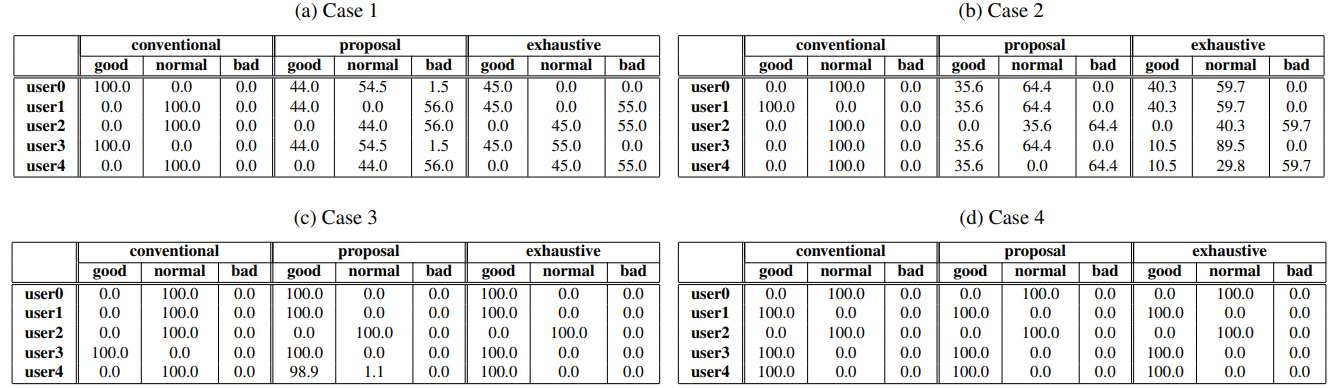Tables 2 and 3 show the evaluation results for user satisfaction. Each value indicates the percentages of time that user satisfaction is good, normal, or bad. As shown in these tables, all users felt normal or good under the conventional method. On the other hand, under the proposed method, some users may have felt bad because the air conditioner setting was raised due to a decrease in the power consumption reward as the outside temperature increased.

Tables 4 and 5 show the achievement rates from device settings and the total reward achievement rates from each method. The achievement rate of a device setting is the rate matching the exhaustive search by each device setting at each time. The TR achievement rate is the ratio of total rewards under each method to the total rewards from the exhaustive search. From these tables, the TR achievement rate under the proposed method is high compared to the conventional method. The average TR rate in Fig. 5 was 68.1% under the conventional method and 99.9% under the proposed method. The average TR rate in Fig. 6 was 67.4% under the conventional method and 99.1% under the proposed method. Therefore, the superiority of the proposed method was verified for all user patterns. Note that although the air conditioner parameters selected under the proposed method and selected under the exhaustive search were different, total reward was almost the same.

##### Fig. 5. Outside temperatures and air conditioner settings each time (${\alpha}$ = 3).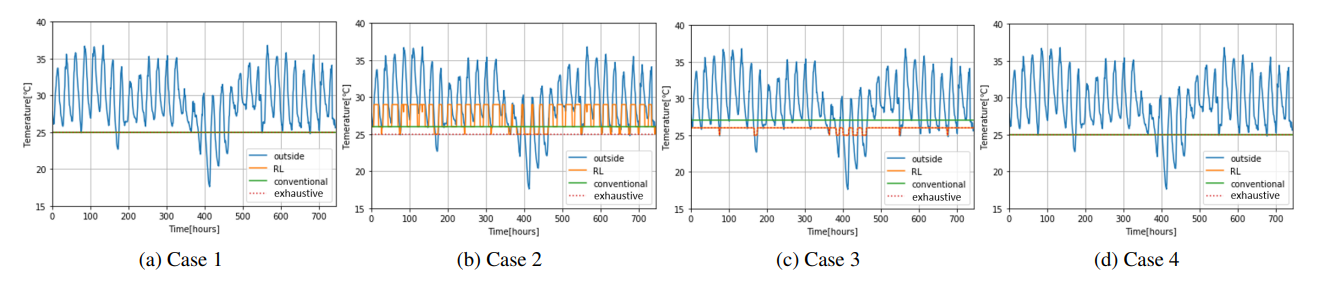##### Fig. 6. Outside temperatures and air conditioner settings each time (${\alpha}$ = 2.5).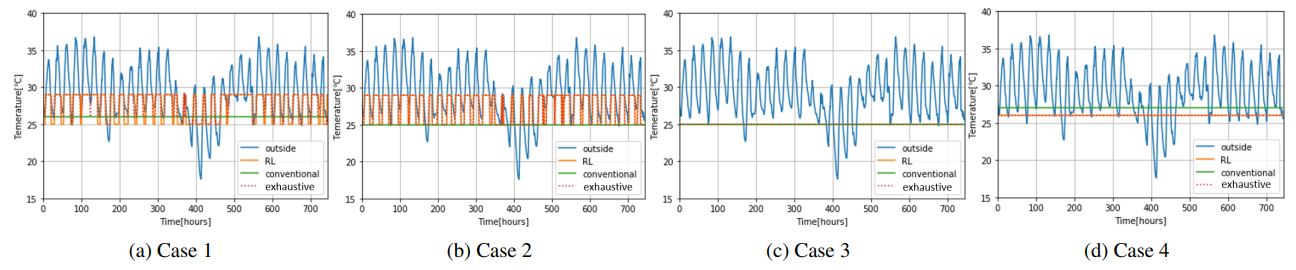##### Table 4. Achievement rates from actuator parameters and total rewards compared with exhaustive search (${\alpha}$ = 3).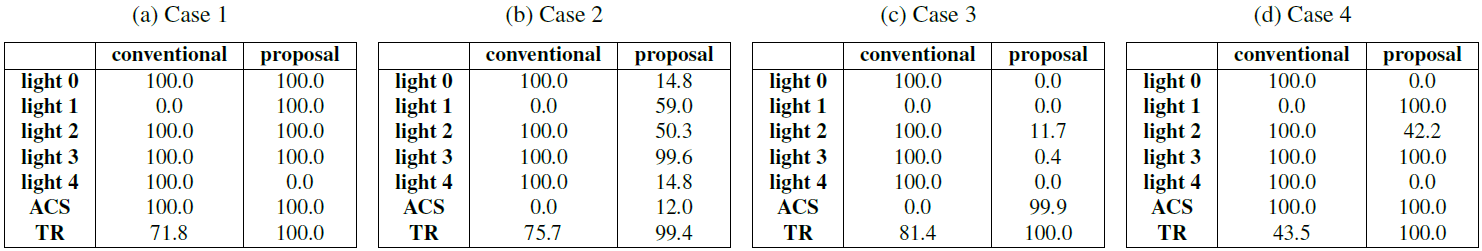##### Table 5. Achievement rates from actuator parameters and total rewards compared with exhaustive search (${\alpha}$ = 2.5).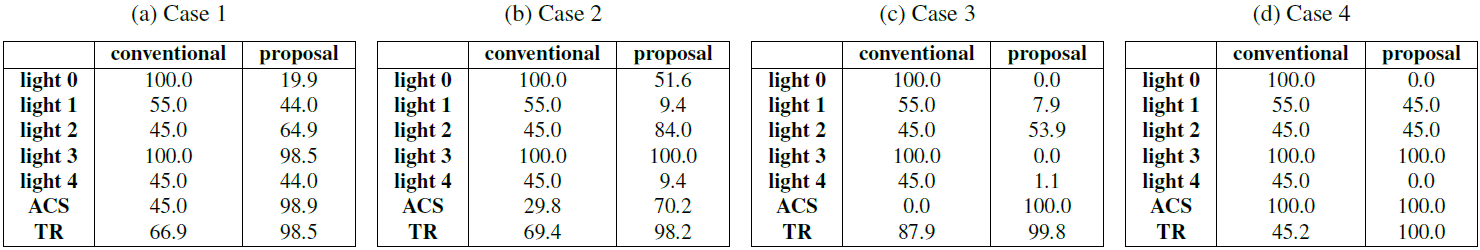Next, we describe the influence from changing weights. From Figs. 5 and 6, the variation ranges of the air conditioner settings are different in some cases. This is because the proprieties of power consumption and user satisfaction are changed by adjusting the weights. The proposed method selects high temperature settings to reduce power consumption when ${\alpha}$ is small, as shown in cases 1, 2, and 3. On the other hand, in Case 4, the air conditioner setting did not change even when weight ${\alpha}$ changed. This is because the penalty for decreasing user satisfaction due to temperature changes is too large to allow changing the setting.

### 4.3 Evaluations when Increasing the Number of Users

Next, we show the evaluation results when there were 10 users. Fig. 7 shows the outside temperatures and air conditioner settings under each method. Tables 6 and 7 show the evaluation results under each method based on the user satisfaction percentage, the achievement rates from actuator parameters, and the total reward. In this evaluation, the results from the exhaustive search cannot be obtained because the calculation overhead is too high. From the results, the proposed method selected parameters that achieved the same reward as the exhaustive search, even when the number of users increased.

##### Fig. 7. Outside temperatures and air conditioner settings versus time with 10 users.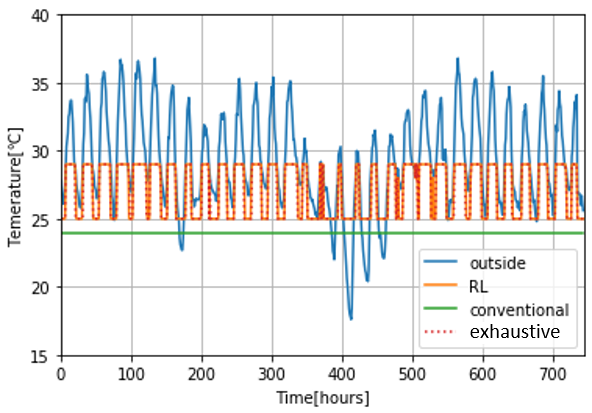##### Table 6. User satisfaction percentage in all periods with 10 users.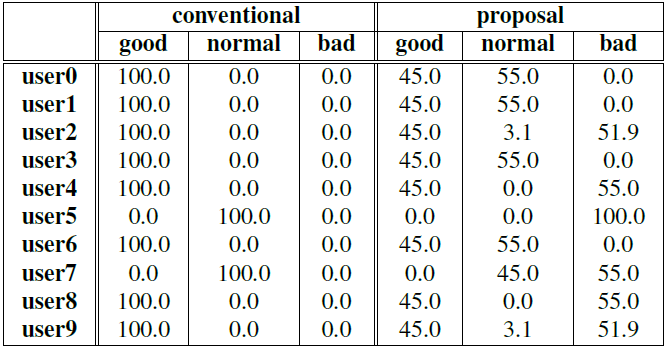##### Table 7. Achievement rates from actuator parameters and the total reward, compared with exhaustive search when there are 10 users.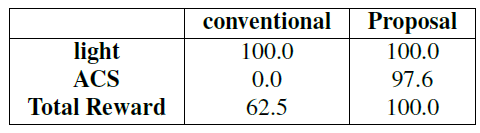## 5. Conclusion

In this study, we proposed a consensus building method to reduce power consumption and increase user satisfaction. The proposed method applies deep reinforcement learning to reduce the calculation overhead. From the evaluation results, we clarified that the proposed method is superior to the conventional method.

In this paper, we did not include a case where the scale of the environment increases, such as increases in the number of users or the amount of control equipment. Therefore, a future task is to verify whether this method can be applied even when the scale of the environment increases. In addition, this control method assumes the room temperature is always the same as the previous temperature setting of the air conditioner. However, considering the user’s position and other external factors, it is necessary to reflect sensor values collected in real time. Therefore, another future task is to improve this method to one that considers more real-time values.

### REFERENCES

1
Levermore G. J., 2000, Building Energy Management Systems: Applications to low-energy HVAC and natural ventilation control., Taylor & Francis2
Zhou B., Li W., Chan K. W., Cao Y., Kuang Y., Liu X., Wang X., 2016, Smart home energy management systems: Concept, configurations, and scheduling strategies, Renewable and Sustainable Energy Reviews, Vol. 61, pp. 30-403
Tarutani Y., Oct. 2018, Proposal of a consensus builder for environmental condition setting in spaces where people with various preferences coexist, in Proceedings of the 9th International conference on ICT convergence (ICTC) 2018, pp. 652-6574
Vimalanathan K., Babu T. R., 2014., The effect of indoor office environment on the work performance, health and well-being of office workers, Journal of environmental health science and engineering, Vol. 12, No. 1, pp. 1135
Wang Z., Tan Y. K., 2013, Illumination control of led systems based on neural network model and energy optimization algorithm, Energy and Buildings, Vol. 62, pp. 514-5216
Schafer A., Kratky K. W., 2006, The effect of colored illumination on heart rate variability, Complementary Medicine Research, Vol. 13, No. 3, pp. 167-1737
Vrijkotte T. G. M., van Doornen L. J. P., de Geus E. J. C., 2000, Effects of work stress on ambulatory blood pressure, heart rate, and heart rate variability, Hypertension, Vol. 35, No. 4, pp. 880-8868
Haak M., Bos S., Panic S., Rothkrantz L., 2009, Detecting stress using eye blinks and brain activity from eeg signals, Proceeding of the 1st driver car interaction and interface (DCII 2008), pp. 35-60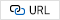9
Jap B. T., Lal S., Fischer P., Bekiaris E., 2009, Using eeg spectral components to assess algorithms for detecting fatigue, Expert Systems with Applications, Vol. 36, No. 2, pp. 2352-235910
Salahuddin L., Cho J., Jeong M. G., Kim D., Aug 2007, Ultra short term analysis of heart rate variability for monitoring mental stress in mobile settings, in 2007 29th Annual International Conference of the IEEE Engineering in Medicine and Biology Society, pp. 4656-465911
Melillo P., Bracale M., Pecchia L., Nov 2011, Nonlinear heart rate variability features for real-life stress detection. case study: students under stress due to university examination, BioMedical Engineering OnLine, Vol. 10, pp. 9612
Begum S., Ahmed M. U., Funk P., Xiong N., von Scheele B., September 2006, Using calibration and fuzzification of cases for improved diagnosis and treatment of stress, in 8th European Conference on Case-based Reasoning workshop proceedings (M. Minor, ed.), pp. 113-12213
van Ravenswaaij-Arts C. M., Kollee L. A., Hopman J. C., Stoelinga G. B., van Geijn H. P., 1993, Heart rate variability, Annals of internal medicine, Vol. 118, No. 6, pp. 436-44714
Japan Meteorological Agency., http://www.jma.go.jp## Author

Yuya TARUTANI received a B.E., an M.E., and a Ph.D. in Information Science and Technology from Osaka University in 2010, 2012, and 2014, respectively. He was an assistant professor in the Cybermedia Center at Osaka University from October 2014 to November 2018. He is currently an assistant professor for the Graduate School of Interdisciplinary Science and Engineering in Health Systems at Okayama University. His research interests include communication networks, design of control methods with IoT devices, and network security in IoT networks. He is a member of the IEICE and the IEEE.

##### Isato OISHI

Isato OISHI received a B.E. and an M.E. in Engineering from Okayama University in 2018 and 2021. His research interests include reinforcement learning.

Yukinobu FUKUSHIMA received a B.E., an M.E., and a Ph.D. from Osaka University, Japan, in 2001, 2003, and 2006, respectively. He is currently an associate professor of the Graduate School of Natural Science and Technology, Okayama University. His research interests include knowledge-defined networking and network virtualization. He is a member of the IEICE, the IEEE, and the ACM.

Tokumi YOKOHIRA received a B.E., an M.E., and a Ph.D. in Information and Computer Sciences from Osaka University, Osaka, Japan, in 1984, 1986, and 1989, respectively. He was an academic at Okayama University from April 1989 to March 2018. Since April 2018, he has been a professor of the Graduate School of Interdisciplinary Science and Engineering in Health Systems at the same university. His current research interests include highly distributed cloud computing environments, designs of virtual networks, technologies to upgrade the speed of the Internet, and technologies to increase fault tolerance on the Internet. He is a member of IEEE Computer and Communication Societies, the Institute of Electronics, Information and Communication Engineers Inc., and the Information Processing Society of Japan.